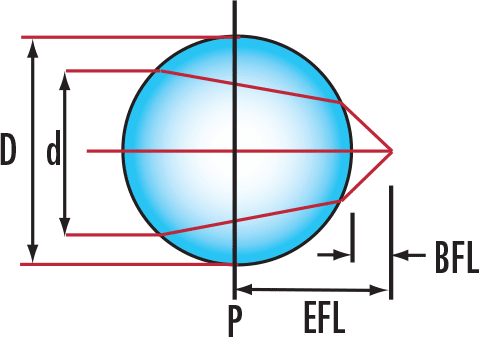# Ball Lens Calculator

View All Technical Tools

### d:

EFL (mm) : --
BFL (mm) : --
NA: --$$\text{EFL} = \frac{n \, D}{4 \left( n - 1 \right)}$$
 $$\text{BFL} = \text{EFL} - \frac{D}{2}$$
 $$\text{NA} = \frac{2 d \left( n - 1 \right) }{n \, D}$$
 EFL Effective Focal Length BFL Back Focal Length NA Numerical Aperture
 n Refractive Index D Diameter of the ball lens d Diameter of the input source

## Description

Determine the effective focal length, the back focal length, and the effective numerical aperture of a ball lens based on its diameter, its refractive index, and the diameter of the collimated beam entering or exiting the ball lens. The effective numerical aperture of the application can only be calculated if the user knows the beam diameter. Knowing the numerical aperture is particularly useful when using ball lenses to couple light from a fiber to a laser or to another fiber.

View All Now